Updating search results...# Grade 7 DLC Blended Math

This collection features resources developed by The Distance Learning Centre (DLC) for blended math in Grade 7.

52 affiliated resources

# Search Resources

View
Selected filters: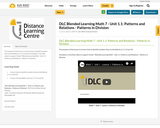Conditional Remix & Share Permitted
CC BY-NC-SA
Rating
0.0 stars

The purpose of this lesson is to learn how to identify numbers that are divisible by 2, 4, 5, 8 and 10.

Included is a YouTube video to support Grade 7 Blended Learning Math - Unit 1.1: Patterns and Relations - Patterns in Division.

Subject:
Math
Material Type:
Activity/Lab
Homework/Assignment
Lesson
Provider:
Sun West Distance Learning Centre (DLC)
12/05/2023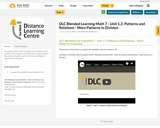Conditional Remix & Share Permitted
CC BY-NC-SA
Rating
0.0 stars

The purpose of this lesson is to explore the divisibility rules of numbers 2-10.

Included is a YouTube video to support Grade 7 Blended Learning Math - Unit 1.2: Patterns and Relations - More Patterns in Division.

Subject:
Math
Material Type:
Activity/Lab
Homework/Assignment
Lesson
Provider:
Sun West Distance Learning Centre (DLC)
12/05/2023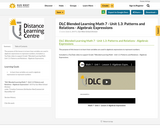Conditional Remix & Share Permitted
CC BY-NC-SA
Rating
0.0 stars

The purpose of this lesson is to learn how variables are used in algebraic expressions to represent numbers.

Included is a YouTube video to support Grade 7 Blended Learning Math - Unit 1.3: Patterns and Relations - Algebraic Expressions.

Subject:
Math
Material Type:
Activity/Lab
Homework/Assignment
Lesson
Provider:
Sun West Distance Learning Centre (DLC)
12/05/2023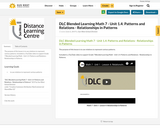Conditional Remix & Share Permitted
CC BY-NC-SA
Rating
0.0 stars

The purpose of this lesson is to use relations to represent various patterns.

Included is a YouTube video to support Grade 7 Blended Learning Math - Unit 1.4: Patterns and Relations - Relationships in Patterns.

Subject:
Math
Material Type:
Activity/Lab
Homework/Assignment
Lesson
Provider:
Sun West Distance Learning Centre (DLC)
12/05/2023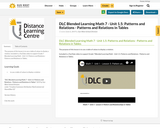Conditional Remix & Share Permitted
CC BY-NC-SA
Rating
0.0 stars

The purpose of this lesson is to use a table of values to display a relation.

Included is a YouTube video to support Grade 7 Blended Learning Math - Unit 1.5: Patterns and Relations - Patterns and Relations in Tables.

Subject:
Math
Material Type:
Activity/Lab
Homework/Assignment
Lesson
Provider:
Sun West Distance Learning Centre (DLC)
12/05/2023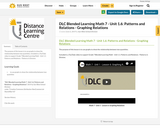Conditional Remix & Share Permitted
CC BY-NC-SA
Rating
0.0 stars

The purpose of this lesson is to use graphs to show the relationship between two quantities.

Included is a YouTube video to support Grade 7 Blended Learning Math - Unit 1.1: Patterns and Relations - Patterns in Division.

Subject:
Math
Material Type:
Activity/Lab
Homework/Assignment
Lesson
Provider:
Sun West Distance Learning Centre (DLC)
12/05/2023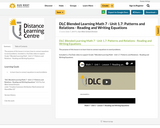Conditional Remix & Share Permitted
CC BY-NC-SA
Rating
0.0 stars

The purpose of this lesson is to learn how to connect equations to word problems.

Included is a YouTube video to support Grade 7 Blended Learning Math - Unit 1.7: Patterns and Relations - Reading and Writing Equations.

Subject:
Math
Material Type:
Activity/Lab
Homework/Assignment
Lesson
Provider:
Sun West Distance Learning Centre (DLC)
12/05/2023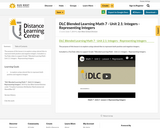Conditional Remix & Share Permitted
CC BY-NC-SA
Rating
0.0 stars

The purpose of this lesson is to explore using colored tiles to represent both positive and negative integers.

Included is a YouTube video to support Grade 7 Blended Learning Math - Unit 2.1: Integers - Representing Integers.

Subject:
Math
Material Type:
Activity/Lab
Homework/Assignment
Lesson
Provider:
Sun West Distance Learning Centre (DLC)
12/05/2023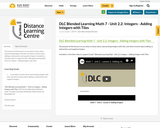Conditional Remix & Share Permitted
CC BY-NC-SA
Rating
0.0 stars

The purpose of this lesson is to use what is known about representing integers with tiles, and what is known about adding, to add positive and negative integers.

Included is a YouTube video to support Grade 7 Blended Learning Math - Unit 2.2: Integers - Adding Integers with Tiles.

Subject:
Arts Education
Drama
Math
Material Type:
Activity/Lab
Homework/Assignment
Lesson
Provider:
Sun West Distance Learning Centre (DLC)
12/05/2023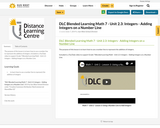Conditional Remix & Share Permitted
CC BY-NC-SA
Rating
0.0 stars

The purpose of this lesson is to learn how to use a number line to represent the addition of integers.

Included is a YouTube video to support Grade 7 Blended Learning Math - Unit 2.3: Integers - Adding Integers on a Number Line.

Subject:
Math
Material Type:
Activity/Lab
Homework/Assignment
Lesson
Provider:
Sun West Distance Learning Centre (DLC)
12/05/2023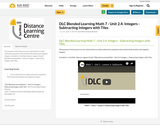Conditional Remix & Share Permitted
CC BY-NC-SA
Rating
0.0 stars

The purpose of this lesson is to use colored tiles to model subtraction equations that involve both positive and negative integers.

Included is a YouTube video to support Grade 7 Blended Learning Math - Unit 2.4: Integers - Subtracting Integers with Tiles.

Subject:
Math
Material Type:
Activity/Lab
Homework/Assignment
Lesson
Provider:
Sun West Distance Learning Centre (DLC)
12/05/2023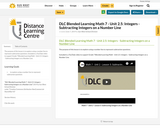Conditional Remix & Share Permitted
CC BY-NC-SA
Rating
0.0 stars

The purpose of this lesson is to explore using a number line to represent subtraction questions.

Included is a YouTube video to support Grade 7 Blended Learning Math - Unit 2.5: Integers - Subtracting Integers on a Number Line.

Subject:
Math
Material Type:
Activity/Lab
Homework/Assignment
Lesson
Provider:
Sun West Distance Learning Centre (DLC)
12/05/2023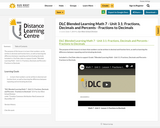Conditional Remix & Share Permitted
CC BY-NC-SA
Rating
0.0 stars

The purpose of this lesson is to learn that numbers can be written in decimal and fraction form, as well as learning the difference between repeating and terminating decimals.

Included is a YouTube video to support Grade 7 Blended Learning Math - Unit 3.1: Fractions, Decimals and Percents - Fractions to Decimals.

Subject:
Math
Material Type:
Activity/Lab
Homework/Assignment
Lesson
Provider:
Sun West Distance Learning Centre (DLC)
12/05/2023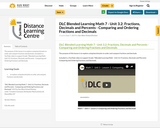Conditional Remix & Share Permitted
CC BY-NC-SA
Rating
0.0 stars

The purpose of this lesson is to explore using benchmarks to order and compare fractions and decimals.

Included is a YouTube video to support Grade 7 Blended Learning Math - Unit 3.2: Fractions, Decimals and Percents - Comparing and Ordering Fractions and Decimals.

Subject:
Math
Material Type:
Activity/Lab
Homework/Assignment
Lesson
Provider:
Sun West Distance Learning Centre (DLC)
12/05/2023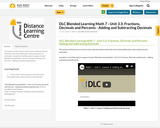Conditional Remix & Share Permitted
CC BY-NC-SA
Rating
0.0 stars

The purpose of this lesson is to learn how to add and subtract decimals to the thousandths place value using from end estimation.

Included is a YouTube video to support Grade 7 Blended Learning Math - Unit 3.3: Fractions, Decimals and Percents - Adding and Subtracting Decimals.

Subject:
Math
Material Type:
Activity/Lab
Homework/Assignment
Lesson
Provider:
Sun West Distance Learning Centre (DLC)
12/05/2023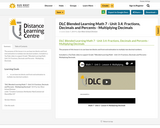Conditional Remix & Share Permitted
CC BY-NC-SA
Rating
0.0 stars

The purpose of this lesson is to use base ten blocks and front end estimation to multiply two decimal numbers.

Included is a YouTube video to support Grade 7 Blended Learning Math - Unit 3.4: Fractions, Decimals and Percents - Multiplying Decimals.

Subject:
Math
Material Type:
Activity/Lab
Homework/Assignment
Lesson
Provider:
Sun West Distance Learning Centre (DLC)
12/05/2023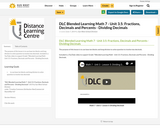Conditional Remix & Share Permitted
CC BY-NC-SA
Rating
0.0 stars

The purpose of this lesson is to use base ten blocks and long division to solve question to involve two decimals.

Included is a YouTube video to support Grade 7 Blended Learning Math - Unit 3.5: Fractions, Decimals and Percents - Dividing Decimals.

Subject:
Math
Material Type:
Activity/Lab
Homework/Assignment
Lesson
Provider:
Sun West Distance Learning Centre (DLC)
12/05/2023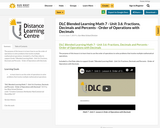Conditional Remix & Share Permitted
CC BY-NC-SA
Rating
0.0 stars

The purpose of this lesson is to learn how to use the order of operations to solve problems that involve multiple mathematical operations.

Included is a YouTube video to support Grade 7 Blended Learning Math - Unit 3.6: Fractions, Decimals and Percents - Order of Operations with Decimals.

Subject:
Math
Material Type:
Activity/Lab
Homework/Assignment
Lesson
Provider:
Sun West Distance Learning Centre (DLC)
12/05/2023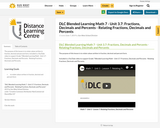Conditional Remix & Share Permitted
CC BY-NC-SA
Rating
0.0 stars

The purpose of this lesson is to relate values written in fraction, decimal and percent form.

Included is a YouTube video to support Grade 7 Blended Learning Math - Unit 3.7: Fractions, Decimals and Percents - Relating Fractions, Decimals and Percents.

Subject:
Machining
Practical & Applied Arts
Material Type:
Activity/Lab
Homework/Assignment
Lesson
Provider:
Sun West Distance Learning Centre (DLC)
12/05/2023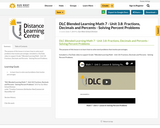Conditional Remix & Share Permitted
CC BY-NC-SA
Rating
0.0 stars

The purpose of this lesson is to learn how to solve word problems that involve percentages.

Included is a YouTube video to support Grade 7 Blended Learning Math - Unit 3.8: Fractions, Decimals and Percents - Solving Percent Problems.

Subject:
Math
Material Type:
Activity/Lab
Homework/Assignment
Lesson
Provider:
Sun West Distance Learning Centre (DLC)